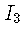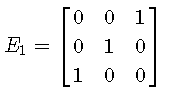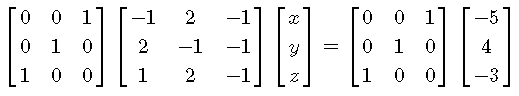# Row Operations and Elementary Matrices

We show that when we perform elementary row operations on systems of equations represented byit is equivalent to multiplying both sides of the equations by an elementary matrix to be defined below.
We consider three row operations involving one single elementary operation at the time. We start with examples and detailed solutions and end up with questions and their solutions.

## Elementary Matrix Equivalent to Interchanging two Rows of a Matrix

We start with the given system in matrix formInterchange rows (1) and (3) and rewrite the system asLet us now consider the identity matrixWe interchange row (1) and (3) into obtain the elementary matrixgiven byand multiply both sides of system (I) byas followsMultiply the matrices, using the associativity on the left side, and simplify towhich is the system of equations (II) obtained above by interchanging rows (1) and (3) in the system (I).
Conclusion : Interchanging rows (1) and (3) is equivalent to multiplying (from the left) the two sides of the system by the elementary matrixobtained from the identity matrix.

## Elementary Matrix Equivalent to Multiplying a Row by a Constant in a Matrix

Let us now consider the system of equations (II) and multiply row (3) by 2 to obtain  
$\begin{bmatrix} 1 & 2 & -1 \\ 2 & -1 & -1 \\ -2 & 4 & - 2 \end{bmatrix} \begin{bmatrix} x \\ y \\ z \end{bmatrix} = \begin{bmatrix} -3 \\ 4 \\ -10 \end{bmatrix} (III)$
Take $$I_3$$ multiply its third row by 2 to obtain the
elementary matrix $$E_2 = \begin{bmatrix} 1 & 0 & 0 \\ 0 & 1 & 0 \\ 0 & 0 & 2 \end{bmatrix}$$ and multiply both sides of the system (II) by $$E_2$$ as follows:
$\begin{bmatrix} 1 & 0 & 0 \\ 0 & 1 & 0 \\ 0 & 0 & 2 \end{bmatrix} \begin{bmatrix} 1 & 2 & -1 \\ 2 & -1 & -1 \\ -1 & 2 & - 1 \end{bmatrix} \begin{bmatrix} x \\ y \\ z \end{bmatrix} = \begin{bmatrix} 1 & 0 & 0 \\ 0 & 1 & 0 \\ 0 & 0 & 2 \end{bmatrix} \begin{bmatrix} -3 \\ 4 \\ -5 \end{bmatrix}$
Use associativity, multiply and simplify the above to obtain
$\begin{bmatrix} 1 & 2 & -1 \\ 2 & -1 & -1 \\ -2 & 4 & - 2 \end{bmatrix} \begin{bmatrix} x \\ y \\ z \end{bmatrix} = \begin{bmatrix} -3 \\ 4 \\ -10 \end{bmatrix}$
which is exactly the system of equations (III).
Conclusion: Multiplying row (3) by 2 is equivalent to multiplying the two sides of the system by the elementary matrix $$E_2 = \begin{bmatrix} 1 & 0 & 0 \\ 0 & 1 & 0 \\ 0 & 0 & 2 \end{bmatrix}$$ obtained from the identity matrix $$I_3$$.

## Elementary Matrix Equivalent to Adding a Multiple of a Row to Another Row in a Matrix

Let us now consider the system of equations (III), multiply row (1) by - 2 add it to row (2) to obtain:
$\begin{bmatrix} 1 & 2 & -1 \\ 0 & - 5 & 1 \\ -2 & 4 & - 2 \end{bmatrix} \begin{bmatrix} x \\ y \\ z \end{bmatrix} = \begin{bmatrix} -3 \\ 10 \\ -10 \end{bmatrix} (IV)$
Take $$I_3$$ multiply its row (1) by - 2 and add it to row (2) to obtain $$E_3 = \begin{bmatrix} 1 & 0 & 0 \\ -2 & 1 & 0 \\ 0 & 0 & 1 \end{bmatrix}$$ and multiply both sides of the system (III) by $$E_3$$ as follows:
$\begin{bmatrix} 1 & 0 & 0 \\ -2 & 1 & 0 \\ 0 & 0 & 1 \end{bmatrix} \begin{bmatrix} 1 & 2 & -1 \\ 2 & -1 & -1 \\ -2 & 4 & - 2 \end{bmatrix} \begin{bmatrix} x \\ y \\ z \end{bmatrix} = \begin{bmatrix} 1 & 0 & 0 \\ -2 & 1 & 0 \\ 0 & 0 & 1 \end{bmatrix} \begin{bmatrix} -3 \\ 4 \\ -10 \end{bmatrix}$
Use associativity, multiply and simplify the above to obtain

$\begin{bmatrix} 1 & 2 & -1 \\ 0 & - 5 & 1 \\ -2 & 4 & - 2 \end{bmatrix} \begin{bmatrix} x \\ y \\ z \end{bmatrix} = \begin{bmatrix} -3 \\ 10 \\ -10 \end{bmatrix}$
which exactly the system of equations (IV).
Conclusion: Add row (1) multiplied by - 2 to row (2) is equivalent to multiplying the two sides of the system by the elementary matrix $$E_3 = \begin{bmatrix} 1 & 0 & 0 \\ -2 & 1 & 0 \\ 0 & 0 & 1 \end{bmatrix}$$ obtained from the identity matrix $$I_3$$.

## Inverse of the Elementary Matrices

One of the advantages in using elementary matrices is that their inverse can be obtained without heavy calculations.

Inverse of Elementary Matrix E1
In the above examples, We obtained the elementary matrix $$E_1$$ by interchanging rows (1) and (3), the inverse of $$E_1$$ is obtained from I3 by interchanging rows (3) and (1); hence the inverse of $$E_1$$ is given by $E_1^{-1} = \begin{bmatrix} 0 & 0 & 1 \\ 0 & 1 & 0 \\ 1 & 0 & 0 \end{bmatrix}$
We now check that
$$E_1 \cdot E_1^{-1} = E_1^{-1} \cdot E_1^ = I_3$$
$E_1 \cdot E_1^{-1} = \begin{bmatrix} 0 & 0 & 1 \\ 0 & 1 & 0 \\ 1 & 0 & 0 \end{bmatrix} \cdot \begin{bmatrix} 0 & 0 & 1 \\ 0 & 1 & 0 \\ 1 & 0 & 0 \end{bmatrix} = \begin{bmatrix} 1 & 0 & 0 \\ 0 & 1 & 0 \\ 0 & 0 & 1 \end{bmatrix}$ There no need to check that $$E_1^{-1} \cdot E_1^ = I_3$$ beacuse $$E_1 = E_1^{-1}$$.

Inverse of Elementary Matrix E2
In the above examples, We obtained the elementary matrix $$E_2$$ by multiplying row (3) by 2, the inverse of $$E_2$$ is obtained from I3 by dividing row (3) by 2; hence the inverse of $$E_2$$ is given by $E_2^{-1} = \begin{bmatrix} 1 & 0 & 0 \\ 0 & 1 & 0 \\ 0 & 0 & 1/2 \end{bmatrix}$
We now check that
$$E_2 \cdot E_2^{-1} = E_2^{-1} \cdot E_2^ = I_3$$
$E_2 \cdot E_2^{-1} = \begin{bmatrix} 1 & 0 & 0 \\ 0 & 1 & 0 \\ 0 & 0 & 2 \end{bmatrix} \cdot \begin{bmatrix} 1 & 0 & 0 \\ 0 & 1 & 0 \\ 0 & 0 & 1/2 \end{bmatrix} = \begin{bmatrix} 1 & 0 & 0 \\ 0 & 1 & 0 \\ 0 & 0 & 1 \end{bmatrix}$
$E_2^{-1} \cdot E_2^ = \begin{bmatrix} 1 & 0 & 0 \\ 0 & 1 & 0 \\ 0 & 0 & 1/2 \end{bmatrix} \cdot \begin{bmatrix} 1 & 0 & 0 \\ 0 & 1 & 0 \\ 0 & 0 & 2 \end{bmatrix} = \begin{bmatrix} 1 & 0 & 0 \\ 0 & 1 & 0 \\ 0 & 0 & 1 \end{bmatrix}$

Inverse of Elementary Matrix E3
In the above examples, We obtained the elementary matrix $$E_3$$ by multiply row (1) by - 2 add it to row (2). The inverse of $$E_3$$ is obtained from I3 by multiplying row (1) by - 2 and subtracting it from row (2); hence the inverse of $$E_3$$ is given by $E_3^{-1} = \begin{bmatrix} 1 & 0 & 0 \\ 2 & 1 & 0 \\ 0 & 0 & 1 \end{bmatrix}$

We now check that
$$E_3 \cdot E_3^{-1} = E_3^{-1} \cdot E_3^ = I_3$$
$E_3 \cdot E_3^{-1} = \begin{bmatrix} 1 & 0 & 0 \\ -2 & 1 & 0 \\ 0 & 0 & 1 \end{bmatrix} \cdot \begin{bmatrix} 1 & 0 & 0 \\ 2 & 1 & 0 \\ 0 & 0 & 1 \end{bmatrix} = \begin{bmatrix} 1 & 0 & 0 \\ 0 & 1 & 0 \\ 0 & 0 & 1 \end{bmatrix}$
$E_3^{-1} \cdot E_3^ = \begin{bmatrix} 1 & 0 & 0 \\ 2 & 1 & 0 \\ 0 & 0 & 1 \end{bmatrix} \cdot \begin{bmatrix} 1 & 0 & 0 \\ -2 & 1 & 0 \\ 0 & 0 & 1 \end{bmatrix} = \begin{bmatrix} 1 & 0 & 0 \\ 0 & 1 & 0 \\ 0 & 0 & 1 \end{bmatrix}$

## Questions on Elementary Matrices

• Part 1
Which of the following is an elementary? Explain. $A = \begin{bmatrix} 0 & 1 \\ 1 & 0\\ \end{bmatrix} ,\quad B = \begin{bmatrix} 1 & 0 & 0 \\ 0 & 0 & 1 \\ 0 & 1 & 0 \\ \end{bmatrix} ,\quad C = \begin{bmatrix} 1 & 0\\ 4 & 1 \end{bmatrix} \\ ,\quad D = \begin{bmatrix} 1 & 0 & 0 \\ 0 & 1 & 0 \\ -5 & 0 & 1 \\ \end{bmatrix} ,\quad E = \begin{bmatrix} 1 & 0 & 0 \\ 1 & 1 & 0 \\ 1 & 0 & 1 \\ \end{bmatrix} ,\quad F = \begin{bmatrix} 1 & 0 & 0 & 0 \\ 0 & 1 & 0 & 0\\ 7 & 0 & 1 & 0\\ 0 & 0 & 0 & 1 \end{bmatrix}$
• Part 2
What is the elementary matrix of the systems of the form $A X = B$ for following row operations?
A) A is 2 by 2 matrix, add 3 times row(1) to row(2)?
B) A is 3 by 3 matrix, multiply row(3) by - 6.
C) A is 5 by 5 matrix, multiply row(2) by 10 and add it to row 3.

• Part 3
Find the inverse to each elementary matrix found in part 2.

## Solutions

• Part 1
A : interchange rows (1) and (2)
B: interchange rows (2) and (3)
C: add 4 times row (1) to row (2)
D: add - 5 times row (1) to row (3)
E: is not an elementary matrix
F: add 7 times row(1) to row (3)
• Part 2
A) $$A = \begin{bmatrix} 1 & 0 \\ 3 & 1\\ \end{bmatrix}$$

B) $$A = \begin{bmatrix} 1 & 0 & 0\\ 0 & 1 & 0\\ 0 & 0 & -6 \end{bmatrix}$$

C) $$A = \begin{bmatrix} 1 & 0 & 0 & 0 & 0\\ 0 & 1 & 0 &0 & 0 \\ 0 & 10 & 1 &0 & 0 \\ 0 & 0 & 0 & 1 & 0 \\ 0 & 0 & 0 & 0 & 1 \end{bmatrix}$$
• Part 3
The inverse of the elementary matrices in part 2.

A) Mulriply row(1) by - 3 times and add it to row(3)
$$A^{-1} = \begin{bmatrix} 1 & 0 \\ -3 & 1\\ \end{bmatrix}$$

B) Divide row (3) by - 6
$$A^{-1} = \begin{bmatrix} 1 & 0 & 0\\ 0 & 1 & 0\\ 0 & 0 & -1/6 \end{bmatrix}$$

C) multiply row(2) by - 10 and add it to row(3).
$$A^{-1} = \begin{bmatrix} 1 & 0 & 0 & 0 & 0\\ 0 & 1 & 0 &0 & 0 \\ 0 & -10 & 1 &0 & 0 \\ 0 & 0 & 0 & 1 & 0 \\ 0 & 0 & 0 & 0 & 1 \end{bmatrix}$$